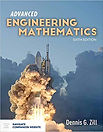top of page# ​• Extreme value theory

• Random fields

• Sampling-based reliability analysis methods including Monte Carlo simulation

• Response surface development

• Random processes

• System reliability

ME 6543 -Machine Learning and  Data Analytics

• Parametric regression: Linear/polynomial regression

• Variable selection: Regularization and cross-validation

• Nonparametric regression: K-nearest neighbor, Gaussian processes

• Classification basics: Loss functions, logistic regression, decision trees

• Classification advanced: Support vector machines

• Ensemble methods: Boosting, bagging, random forest

• Dimension reduction: Principal component analysis

• Clustering: K-means clustering

• Deep learning: Feedforward neural networks, convolutional neural networks

• Reinforcement Learning: Markov decision process

ME 5013 - Advanced Data Analytics

• Data visualization: Multivariate, hierarchical, temporal, and network data visualization

• Regression and regularization: Linear regression, logistic regression, regularization, ridge regression, nonparametric regression with Gaussian processes

•  Classification basics: Loss functions, naive Bayes, linear classifiers

• Support vector machines, convex optimization

• Kernels: Model selection, cross validation

• Ensemble methods: Boosting, bagging, random forest

• Dimension reduction: principal component analysis

• Clustering, mixture models, EM algorithms

• Bayesian Inference, sampling algorithms, MCMC

• Stochastic processes: Markov models, hidden Markov models

• Graphical Models: State space models, Kalman filter

EGR 5213 Introduction to Modelling and Simulation

• Preliminaries

• Random variable generation

• Markov Chain

• Monte Carlo Methods

• Monte Carlo Integration

• Monte Carlo Optimization

• Markov Chain Monte Carlo

• Convergence

• Discrete Event Simulation

• Simulation concepts

• Modeling basic operations and inputs

• Statistical analysis of output

• Continuous and combined models

• Simulation Software

• Excel

• ARENA

ME 4723 – Reliability and Quality Control

• Reliability concepts

• Probability and life distribution for reliability analysis

• Design for six sigma

• Product development

• Failure modes, mechanisms, and effect analysis

• Probabilistic design for reliability and the factor of safety

• Reliability estimation techniques

• Process control and process capability

• Analyzing product failures and root causes

•  System reliability modeling

• Warranty analysis

ME 3263 – Manufacturing Engineering

• Introduction to Manufacturing Engineering: Products, Processes, and Systems

• Manufacturing Systems: An Overview of Basics

• Mechanical Properties of Materials

• Tolerances

• Measurement and Quality Assurance

• Manufacturing Processes

EGR 2323 – Applied Engineering Analysis I

• Mathematical modeling of engineering problems

• Separable ODE

•  Integrating factors

• First-, second-, and higher-order linear constant coefficient ODE’s

• Non-homogeneous ODE

• Laplace Transforms

• s- and t- translation

• Convolution Solution of an ODE via Laplace transform

• Existence and uniqueness of solution to a system of linear algebraic equations

• Gauss elimination and rank

• Determinant, Cramer’s rule, and inverse of a matrix

• Eigenvalues and eigenvectors

• Diagonalization

• Solutions to system of ODEbottom of page